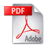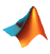(Finite Element Analysis)

This is a FEM project on Tehran Telecommunication Tower. The analysis is simplified with some assumptions and it's just for the top part of the tower.

According to symmetry the structure of the top part simplified to a small one. After that a MATLAB code has been written to create and assemble the stiffness matrixes of elements and the global stiffness matrix. At the end the matrix equation has been solved for the unkowns.

 This is the report of the project in persian:And the code written in MATLAB:___________________________________